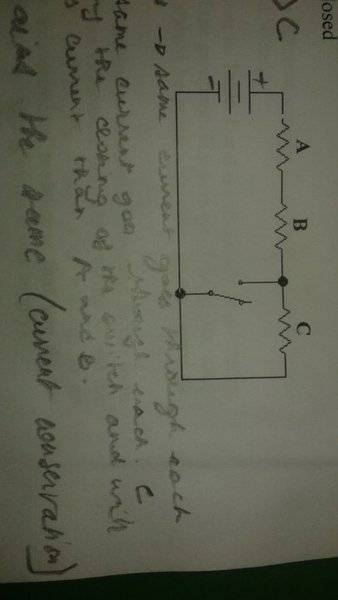# What happens to the total power dissipated in the circuit ?

## Homework Statement

What happens to the total power dissipated in the circuit once the switch is closed?## Homework Equations

Pr=Pbat=dEth/dt=(dq/dt)*Vr=I*Vr[/B]

## The Attempt at a Solution

V total and I toral remain the same so the power dissipated by the circuit stays the same...?

phyzguy
Why do you think Itotal remains the same? What is the resistance across the battery with the switch open and with it closed?

gneill
Mentor
Can you show some equations to back up your guess?

Why do you think Itotal remains the same? What is the resistance across the battery with the switch open and with it closed?

Well....for the current, it's current conservation. Switch open: I total goes through A, B and C ( they're in series) and then goes back to the battery. Switch closed: I total goes through A then B then splits into let's say I1( through the middle resustless wire) and I2 ( through C) at the upper junction. Then it becomes I total again at the lower junction. I think the closed switch short-circuits C but I am not sure this means I2 is null....As for the voltages, I think the voltage across C has to decrease once the switch is closed ( V=IR, I decreases; V decreases). But the total voltage has to stay the same ( equal to the voltage provided by the battery) so the voltages across A and B have to increase...but that would mean that the current through A and B increases.....?!?

Can you show some equations to back up your guess?

Pr=Pbat=dEth/dt=(dq/dt)*Vr=I*Vr
I got his from my textbook. We haven't learned it in class yet, so I am confused about it and the textbook does not help..

cnh1995
Homework Helper
Gold Member
What happens to the total resistance of the circuit when the switch is closed?

cnh1995
Homework Helper
Gold Member
But the total voltage has to stay the same ( equal to the voltage provided by the battery) so the voltages across A and B have to increase...but that would mean that the current through A and B increases.....?!?
Right.

Right.
But how is that possible??? A and B are right after the positive terminal of the battery so no matter the situation they should receive I total...?

cnh1995
Homework Helper
Gold Member
But how is that possible??? A and B are right after the positive terminal of the battery so no matter the situation they should receive I total...?
What happens to the total resistance of the circuit when the switch is closed?

phyzguy
But how is that possible??? A and B are right after the positive terminal of the battery so no matter the situation they should receive I total...?

Of course A and B receive all of Itotal, but Itotal is different when the switch is closed than when it is open.

cnh1995
Homework Helper
Gold Member
Switch closed: Req is smaller than 3R
Yes.
C is now in parallel with A and B).
No. C is eliminated from the circuit. Look up the term "short circuit".
So I is smaller when the switch is closed.
Are you sure? Equivalent resistance decreased after closing the switch.

cnh1995
Homework Helper
Gold Member
Itotal=Vtotal*Req.
I=V/R and not V*R.

I=V/R and not V*R.
True! So the current increases.